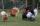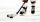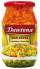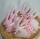Have solution

The sum of four consecutive even numbers is 96. Determine these numbers.

Result

a =  21
b =  23
c =  25
d =  27

Solution:

a+b+c+d=96
b=a+2
c=b+2
d=c+2

a+b+c+d = 96
a-b = -2
b-c = -2
c-d = -2

a = 21
b = 23
c = 25
d = 27

Calculated by our linear equations calculator.

Leave us a comment of example and its solution (i.e. if it is still somewhat unclear...):Be the first to comment!To solve this example are needed these knowledge from mathematics:

Do you have a linear equation or system of equations and looking for its solution? Or do you have quadratic equation?

Next similar examples:

1. Street numbersLada came to aunt. On the way he noticed that the houses on the left side of the street have odd numbers on the right side and even numbers. The street where he lives aunt, there are 5 houses with an even number, which contains at least one digit number 6.
2. BirdsOn the farm they have a total of 110 birds. Geese and turkeys together is 47. Hens is three times more than the turkey. How much is poultry by species?
3. Brothers and sistersLenka has as many brothers as sisters. Her brothers have twice as many sisters as brothers. How many brothers and sisters are in the family?
4. Dropped sheetsThree consecutive sheets dropped from the book. The sum of the numbers on the pages of the dropped sheets is 273. What number has the last page of the dropped sheets?
5. Hockey teams goalsHockey teams fired 200 goals. The second team 13 less than first team, third 16 less than the first and fourth tean 19 goals less than first. How many goals fired each team?
6. The storeThe store received the same number of cans of peas and corn. The first day sold 10 cans of peas and 166 cans ofcorn so that left 5 times more peas than corn cans. How many cans of each kind were in the store?
7. Unknown number 11That number increased by three equals three times itself?
8. Forest nurseryIn the forest nursery after winter, they found that 1/10 stems died out of them. For them, they land 193 new spruces. How many spruces are in the forest nursery?
9. Find xSolve: if 2(x-1)=14, then x= (solve an equation with one unknown)
10. Simple equationSolve for x: 3(x + 2) = x - 18
11. InfirmaryTwo thirds of children from the infirmary went on a trip seventh went to bathe and 40 children remained in the gym. How many children were treated in the infirmary?
12. Negative in equation2x + 3 + 7x = – 24, what is the value of x?
13. Simple equationSolve the following simple equation: 2. (4x + 3) = 2-5. (1-x)
14. NormThree workers planted 3555 seedlings of tomatoes in one dey. First worked at the standard norm, the second planted 120 seedlings more and the third 135 seedlings more than the first worker. How many seedlings were standard norm?
15. Tickets 3A total of 645 tickets were sold for the school play. They were either adult tickets or student tickets. There were 55 fewer student tickets sold than adult tickets. How many adult tickets were sold?
16. Equation 29Solve next equation: 2 ( 2x + 3 ) = 8 ( 1 - x) -5 ( x -2 )
17. Chicken and rabbits (classic)Grandmother breeding chickens and rabbits. It found that the yard has 35 heads and 108 legs. How many chickens and rabbits breeding in the yard?